• 翻译文件，On-Line Parameter Identification Algorithms Based on Householder Transformation
• 这种方式无需改变三角形侧TA配置，直接利用三角形侧线电流构建了变压器绕组的电阻和电感参数辨识算法，利用带动态遗忘因子的最小二乘法，消除了最小二乘法“数据饱和”的影响．应用EMTP进行了仿真，结果表明，参数...
• 以块脉冲函数为基函数，利用正交函数变换将由偏微分方程描述的分布参数系统模型转化为最小二乘形式的代数方程，在此基础上推导出大型立式淬火炉温度分布参数系统的参数辨识算法，并进行仿真和31m立式淬火炉温度控制...
• 为此,本文研究了一种有输入扰动的的逆 系统无偏参数辨识算法,该算法先通过小波变换估计输入信号噪声的方差,再由估计得到的方差,通过偏差消除的 递推最小二乘法,对逆系统的参数进行无偏辨识.该算法降低了对输入辨识...
• 为了辨识一类非线性Hammerstein-Wiener系统，基于递推贝叶斯算法和奇异值分解，提出了一种两阶段在线辨识算法。该算法首先利用递推贝叶斯算法估计乘积项参数，然后利用奇异值分解得到待估计参数。仿真结果表明，所提...参数估计 模块化系统 两阶段算法 递推贝叶斯算法 奇异值分解
• 以M-K(伯格斯)蠕变模型为例,给出蠕变模型参数辨识方法,并编写Matlab算法函数通过COM组件从而剥离出程序,借助VB环境下编制面向对象的可视化计算软件。结果表明,该软件用于岩石蠕变模型参数辨识的计算过程同样有较好的...
• 针对初值和噪声统计特性未知情形下的飞行器系统辨识的问题，提出了基于期望最大化( expectation maximization，EM) 和求容积卡尔曼平滑器( cubature Kalman smoother，CKS) 的辨识算法。该算法用期望最大化算法对...研究论文
• 进化粒子群优化算法在船舶纵向运动参数辨识算法设计中的应用.pdf
• 以M-K(伯格斯)蠕变模型为例,给出蠕变模型参数辨识方法,并编写Matlab算法函数通过COM组件从而剥离出程序,借助VB环境下编制面向对象的可视化计算软件。结果表明,该软件用于岩石蠕变模型参数辨识的计算过程同样有较好的...
• 针对频域子空间算法在模态参数识别中的使用限制，提出了一种分段计算的频域子空间模态参数辨识方法。该方法对输入和输出的频域数据进行分段，再重新计算广义算子，构成新的状态方程，从而实现模态参数辨识。对该方法...
• 通过Gauss滤波和Hilbert变换相结合...应用该方法，完成了空间柔性桁架结构的模态参数辨识，得到了比传统处理方法更接近理论计算结果的结构模态参数，能够有效地避免柔性结构参数辨识中的漏频现象，并具有高的辨识精度。
• 基于动态模块的伺服系统联合仿真与参数辨识算法研究研究论文
• 为了在有色噪声干扰情况下获得无偏估计，基于辅助模型思想和分解技术，提出了一种带协方差重置的两阶段递推贝叶斯辨识算法。该算法首先把待辨识模型分解成两个虚拟子模型，然后分别辨识；同时，把估计到的噪声方差...
• 介绍了基于递推最小二乘法进行系统辨识的基本原理，对给定的实际输入输出数据运用MATLAB的M语言编写递推最小二乘算法，最后给出相应的仿真结果和分析，并对得到的模型进行验证。

第一次实验利用5组数据，根据LS算法得出 θ 和P，此第6组开始递推。

theta=zeros(400,4);
p=zeros(20,4);
p(1,:)=[1 1 1 1];
p(2,:)=[1 1 1 1];
k=3;
while(k<21)
p(k,1)=-ym(k-1);
p(k,1)=-ym(k-1);
p(k,2)=-ym(k-2);
p(k,3)=um(k-1);
p(k,4)=um(k-2);
k=k+1;
end
xi=zeros(400,1);
Pm=inv(p'*p);
thetam=Pm*p'*ym;
while(k<400)
fai=[-y(k-1) -y(k-2) u(k-1) u(k-2)]';
xi(k)=y(k)-fai'*thetam;
G=Pm*fai/(1+fai'*Pm*fai);
Pm=Pm-G*fai'*Pm;
thetam=thetam+G*(y(k)-fai'*thetam);
theta(k,:)=thetam';
k=k+1;
end
figure,plot([1:400],theta);
xlabel('试验次数');ylabel('参数估计');
legend('a_1','a_2','b_1','b_2');
figure,plot([1:400],xi);
xlabel('试验次数');ylabel('残差');

Theta :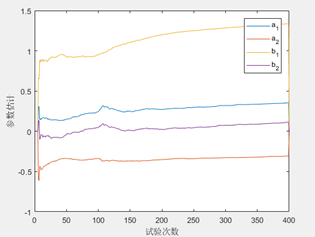残差：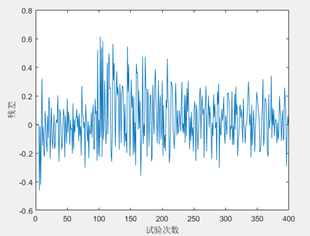运行结果如上图所示，RLS算法在大概50组数据的时候能够实现初步收敛，但是仍然残差较大且且一直处于微小的振荡过程中。特别是到了递推到了100组数据后，残差值明显增大尖峰值甚至达到了0.6，b1曲线收敛的速度缓慢。

初始推测是递推之间的离线辨识数据量不足，将离线辨识的数据量增大四倍到20组。

实验利用20组数据，根据LS算法得出 θ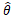和P，此第21组开始递推。再次实现得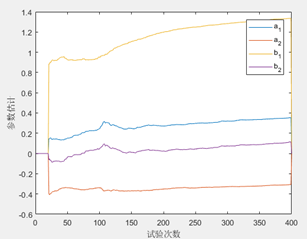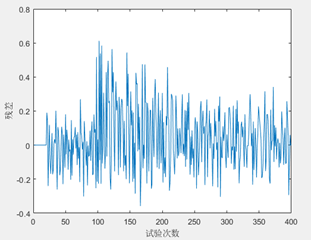从实验的结果来看，在离线辨识的数据量增大能够保证估算精度后在此递推，仍然是存在误差较大且小幅振荡的现象。递推到100组之后，残差明显增大，尖峰值过大的问题仍然没有解决。

第二次实验尝试采用初值设定的方法，即将θ=0，任取P0=σ2I2n*2n其中的I为2n*2n的单位阵，σ特别大，一般在10000-1000000之间。

theta=zeros(400,4);
k=3;
xi=zeros(400,1);
Pm=eye(4,4)*(10^1)^2;
thetam=zeros(4,1);
while(k<401)
fai=[-y(k-1) -y(k-2) u(k-1) u(k-2)]';
xi(k)=y(k)-fai'*thetam;
G=Pm*fai/(1+fai'*Pm*fai);
Pm=Pm-G*fai'*Pm;
thetam=thetam+G*(y(k)-fai'*thetam);
theta(k,:)=thetam';
k=k+1;
end
figure,plot([1:400],theta);
xlabel('试验次数');ylabel('参数估计');
legend('a_1','a_2','b_1','b_2');
figure,plot([1:400],xi);
xlabel('试验次数');ylabel('残差');

当σ= 100000时：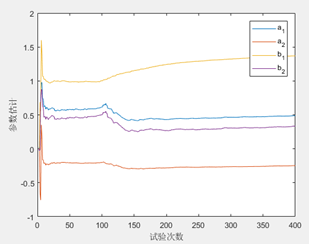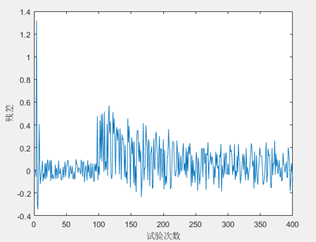运行结果如上图所示，可以看出大约在15组数据后，结果就收敛了，且100组之前的残差值较小，这和LS+RLS算法相比收敛速度得到了明显得上升，但系统在100组数据的时候，仍然发生了参数变化，辨识结果在150组数据左右再次达到收敛。虽然最终都达到了收敛，但是从右边的残差图可以看出，误差的峰值仍然较大，100组数据之后残差值得振荡仍然明显存在。

考虑更改σ之后，再次观察实验效果，

σ= 1000时：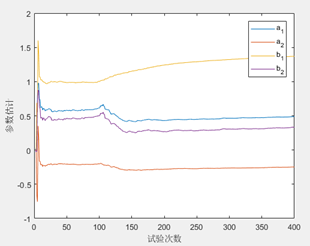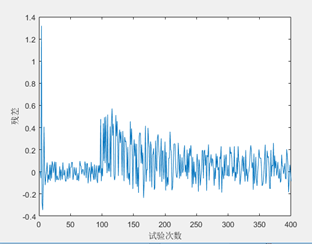当σ= 10时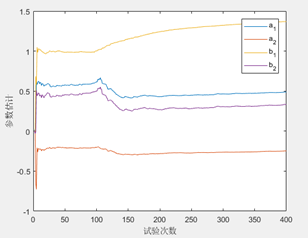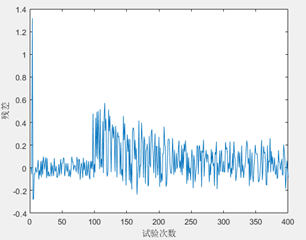从以上实验结果可知，σ对估算性能影响不明显，排除σ取值不准带来的问题。

在对上述实验结果的观察中发现，随着数据采集量的增加，新数据提供的信息被旧的数据掩埋，而辨识算法如果对新旧数据用相同计算度对待，旧的数据就会持续对算法进行影响，这将导致算法修正能力逐步降低，从而无法辨识准确的参数，如果我们能够实现对新旧数据的处理区分开来，对新数据的计算权重更大，对旧数据的计算权重降低，就可以解决旧数据对算法修正能力的影响。

第三次实验在采用初值设定的RLS算法基础上，采用遗忘算法来对参数进行辨识

遗忘算法的原理分析：

在模型不变的基础上，采用初值设定的RLS算法基础上，对新旧信息加上一个计算权重，对新旧信息进行区别处理，增加新信息在算法中的权重，减小旧信息的权重。

算法公式如下：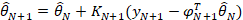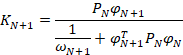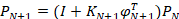其中 p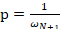是遗忘因子，从式子中可以看出，遗忘因子越小，旧的数据的权重越小，新的数据的权重越大。

theta=zeros(400,4);
p=0.85;
k=3;
Pm=eye(4,4)*(10^1)^2;
thetam=zeros(4,1);
while(k<401)
fai=[-y(k-1) -y(k-2) u(k-1) u(k-2)]';
G=Pm*fai/(p+fai'*Pm*fai);
Pm=(Pm-G*fai'*Pm)/p;
xi(k)=y(k)-fai'*thetam;
thetam=thetam+G*(y(k)-fai'*thetam);
theta(k,:)=thetam';
k=k+1;
end
figure,plot([1:400],theta);
xlabel('试验次数');ylabel('参数估计');
legend('a_1','a_2','b_1','b_2');
figure,plot([1:400],xi);
xlabel('试验次数');ylabel('残差');

当 p = 0.9 时，实验结果如下：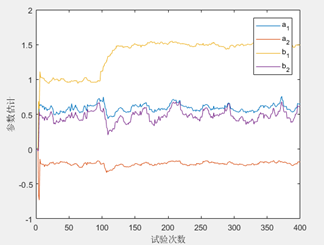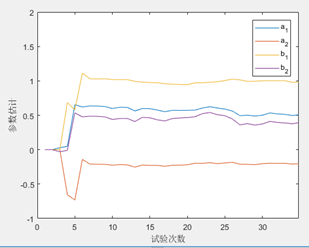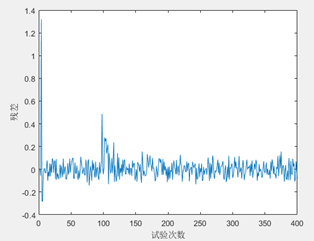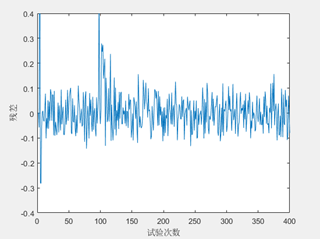根据遗忘算法的实验结果，可以看出初始阶段，能够在5组数据左右就能实现收敛，这比初值设定的RLS算法要快很多；且算法在100组数据之后，残差值能够快速的减小，振荡幅度小且能实现快速收敛。但有a1a2b1b2的波形有明显的振荡，与初值设定的RLS算法相比，此时100组之后由于数据饱和带来的估计误差过大的问题已经基本解决。

再次尝试改变p值之后，算法的效果

P =0.95时，实验结果如下：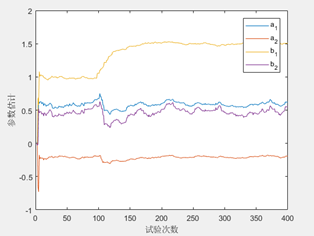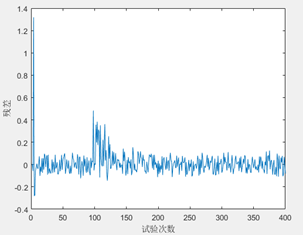P=0.99时，实验结果如下;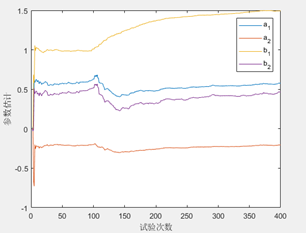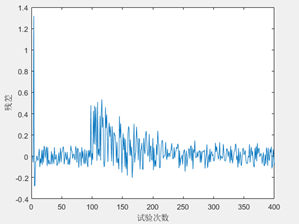P=0.85，实验结果如下：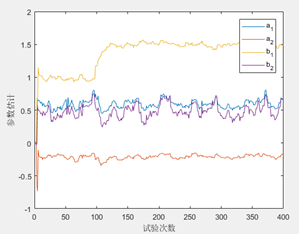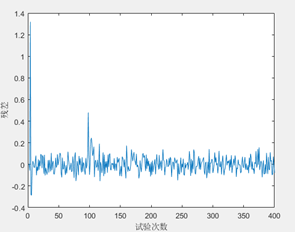从上述三次实验的结果可以看出，随着遗忘因子p的增大，旧数据的权重越大，新数据的权重越小，theta收敛得越慢，辨识参数振荡也更小，辨识结果的收敛性好，但残差波动较大，追踪能力差；遗忘因子p越小，旧数据的权重越小，新数据的权重越大，收敛的速度越快，追踪能力好，残差的波动较小，但也正是由于新数据的权重变大，所以辨识的参数波动也较大，辨识结果的收敛性较差。

在此有此思考，如果我们能够综合p在较大时的收敛性和p在较小时候的快速追踪性，那算法的性能就能得到很大的提升。这在单个遗传因子参数的情况下是无法实现的，我们可以考虑在使算法在不同的情况下使用不同的p，即变p的策略来综合收敛性和快速追踪性能。

第四次实验是根据单遗忘因子所作的改进实验，使用变p的策略来进行参数辨识。

变p算法就是给算法一个定量的参考值，根据这个参考值的大小来调节p的大小，当发现模型的这个指标大于这个参考值时，选择较对应大小的遗传因子。从上节实验中可以看出p=0.99，算法具有较好的收敛特性，曲线较为平滑，缺陷在于到100组数据的时候，对于无法快速的跟随，如果在这个时候将P降低，就可以在残差较大的时候快速跟随，残差较小的时候回到p=0.99，使得算法的收敛性能提升。

theta=zeros(400,4);
k=3;
Pm=eye(4,4)*(10^1)^2;
thetam=zeros(4,1);
while(k<401)
fai=[-y(k-1) -y(k-2) u(k-1) u(k-2)]';
xi(k)=y(k)-fai'*thetam;
p=0.99;
if xi(k)>0.15
p=0.2
end

G=Pm*fai/(p+fai'*Pm*fai);
Pm=(Pm-G*fai'*Pm)/p;
xi(k)=y(k)-fai'*thetam;
thetam=thetam+G*(y(k)-fai'*thetam);
theta(k,:)=thetam';
k=k+1;
end
figure,plot([1:400],theta);
xlabel('试验次数');ylabel('参数估计');
legend('a_1','a_2','b_1','b_2');
figure,plot([1:400],xi);
xlabel('试验次数');ylabel('残差');

变p算法实验结果：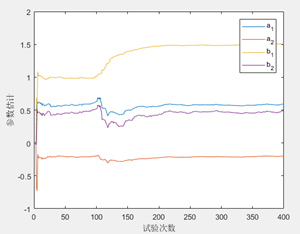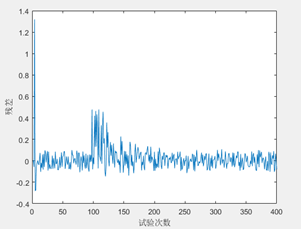从图中可以看出，相比单值p=0.85，在系统的收敛特性得到很大提升，曲线光滑并且没有振荡，相比单值p=0.99，算法的残差明显更小，系统跟随特性也得到了很大提升，基本综合了p=0.85和p=0.99的优点。

此时只是初步调节结果，若试验不同的转换p值，得出最优组合。

当根据参考值p=0.99 和 p=0.7之间转换时实验结果：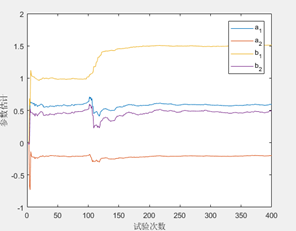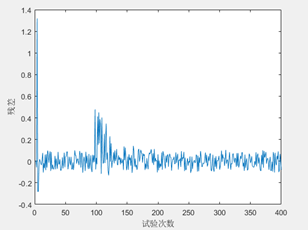当根据参考值p=0.99 和 p=0.5之间转换时实验结果：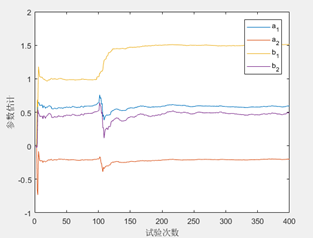当根据参考值p=0.99 和 p=0.3之间转换时实验结果：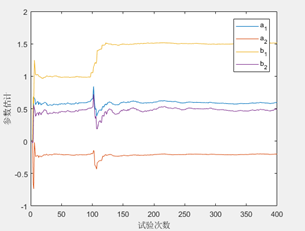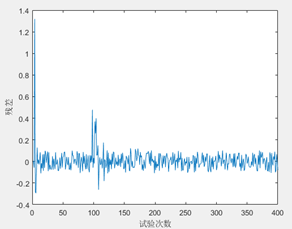经过尝试多组实验，p转换值在p=0.99 和 p=0.3之间算法性能最佳，算法的收敛性和跟随性能都较为优良。

第五次实验 尝试变P法，当残差和大于限定条件时重置P矩阵

theta=zeros(400,4);
k=3;
p=1;
Pm=eye(4,4)*(10^1)^5;
thetam=zeros(4,1);
while(k<401)
fai=[-y(k-1) -y(k-2) u(k-1) u(k-2)]';
xi(k)=y(k)-fai'*thetam;
if xi(k)^2>4*var(xi(1:k))
Pm=eye(4,4)*10^5;
end
G=Pm*fai/(p+fai'*Pm*fai);
Pm=(Pm-G*fai'*Pm)/p;
xi(k)=y(k)-fai'*thetam;
thetam=thetam+G*(y(k)-fai'*thetam);
theta(k,:)=thetam';
k=k+1;
end
figure,plot([1:400],theta);
xlabel('试验次数');ylabel('参数估计');
legend('a_1','a_2','b_1','b_2');
figure,plot([1:400],xi);
xlabel('试验次数');ylabel('残差');

实验结果如下：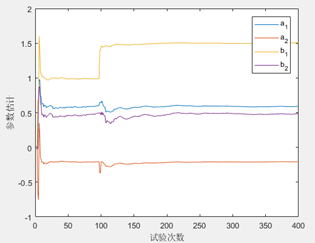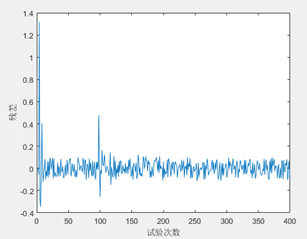其实原理和变p算法相类似，都是基于一个参考条件来重新调整，变P算法是在误差大于一定值时，直接重置P矩阵，由重置的矩阵重新开始递推，就直接把旧的数据舍弃了。

从实验的结果来看，辨识结果在参数变化后的瞬间将旧数据全部抛弃，可以理解为权重为零，重置P矩阵，由新的矩阵开始递推，这样能够有效的减少旧数据对算法的干扰，且不会像遗忘算法一样会导致收敛性能差，导致振荡。同时还具有较好的跟随性能，相当于参数变化之后，用重置的P矩阵再次递推，跟随性能较好。

展开全文参数辨识
• 所以最后，进行估计得到的参数为： 我们的程序设计中，按照论文中的说明进行设计，对于非连续时间的函数方程式： 普罗尼算法步骤： 3）特征解求取： 由式递推求解 2.部分程序： ...

1.问题描述：

建立如下被测信号：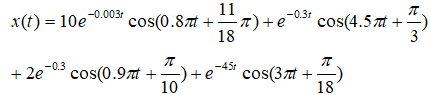被测信号中包含四个振荡模态，在数据窗宽度同样为10s的前提下，利用不同的采样频率做普罗尼计算。根据公式的基本表达式：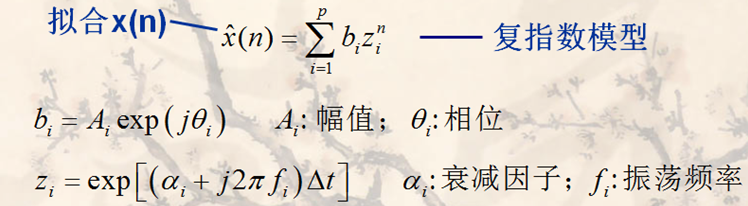所以最后，进行估计得到的参数为：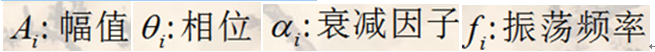我们的程序设计中，按照论文中的说明进行设计，对于非连续时间的函数方程式：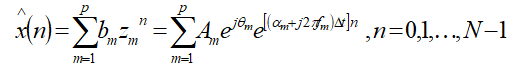普罗尼算法步骤：

展开全文prony算法 参数辨识
• 通过粒子群算法（PSO）可实现多自由度系统二阶动力学方程的参数辨识
• 针对当前大部分光伏（photovoltaic，PV）模型参数辨识算法均存在准确性低和可靠性差等问题，提出了一种采用改进型共生生物搜索算法（symbiotic organisms search，SOS）的光伏组件模型参数辨识方法。首先，为提高...
• 基于prony算法的参数辨识算法的仿真的详细版欢迎订阅本博： https://blog.csdn.net/ccsss22/article/details/115358232 1.问题描述： 建立如下被测信号： 被测信号中包含四个振荡模态，在数据窗宽度同样为10s...

1.问题描述：

建立如下被测信号：被测信号中包含四个振荡模态，在数据窗宽度同样为10s的前提下，利用不同的采样频率做普罗尼计算。

2.部分程序：

function X = func_Prony(Signal,dt);

s     = Signal;
L     = length(s(1:length(Signal)));
Order = ceil(L/2);
R     = [];
K1    = 0;
K2    = 0;

%扩展矩阵
while K1 <= Order
K2  = 1;
Re = [];
while K2 <= Order
u  = Order - K2 +1;
v  = L - K2;
m  = Order - K1 +1;
l  = L - K1;
r  = sum(s(u:v).*conj(s(m:l)));
Re = [Re,r];
K2  = K2 + 1;
end
R = [R,Re'];
K1 = K1 + 1;
end

%计算阶数
Order = func_Order(R);

%计算相关参数
K2 = Order-1;
Re = R(2:end,2:K2+1);
b  = R(:,1);
b  = b(2:end);
a  = pinv(Re)*(-b);

R1 = R(1,:);
R1 = R1(1:K2+1);
a1 = [1 a'];

Ep = sum(R1.*a1);
P  = [1 a'];
z  = roots(P);

%估计序列X
ks    = 1:K2;
X(ks) = s(ks);

for Order = K2+1 : L
Lij      = 1:K2;
X(Order) = sum(-a'.*s(Order-Lij));
Order    = Order+1;
end

Zh = [];
for mm = 0:L-1
Zh = [Zh,z.^mm];
end

Z    = Zh';
Z    = conj(Z);
Zhh  = Z';
b    = (Zhh*Z)^(-1)*Zhh*X';
%最后得到的四个参数值
A     = abs(b)
f     = angle(z)/2/pi/0.001
a     = log(abs(z))/dt
theta = angle(b)/2/pi/dt

3.仿真结论：
注意，这里论文中你所给的那个公式，貌似有点小错误，这里我们使用了两组公式进行计算，一组是你所提供的公式，一组是我们给的测试数据。

仿真结果如下所示：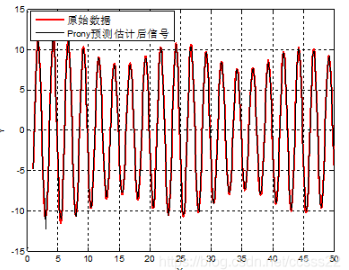A-27-6

展开全文• 基于LCMV的参数辨识算法

千次阅读 2020-10-16 16:39:40
这里的思想主要是广义旁瓣相消器是LCMV的一种等效的...一：一个是采用GSC算法进行降序之后，获得的降秩性能更优，具体可看GSC的相关理论； 二：第二是，这里采用Wq补偿的方式，在实际中，由于直接使用W降秩矩阵的...

这里的思想主要是广义旁瓣相消器是LCMV的一种等效的结构。其主要涉及到的公式有：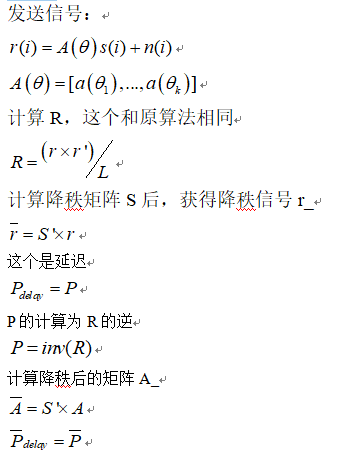这个降秩矩阵S的计算公式，和原论文相同。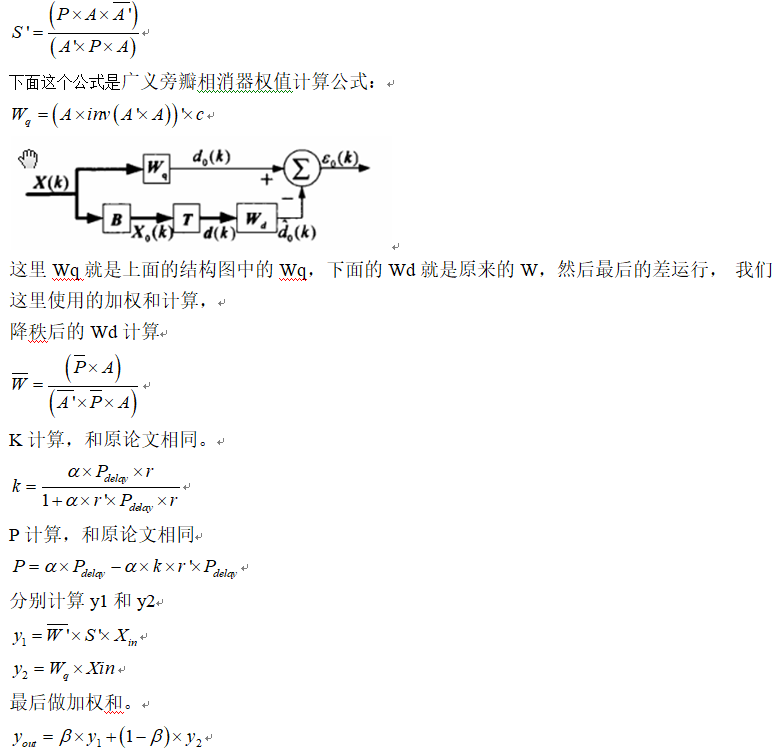首先介绍一下，为什么性能会提高，

这个，有的时候也说不准，这么做一定会提示性能，我们多半都是在理论上说得通的前提下，通过仿真去分析性能，然后来完善理论，这里我弄完这个部分之后，提升性能的原因有二：

一：一个是采用GSC算法进行降序之后，获得的降秩性能更优，具体可看GSC的相关理论；

二：第二是，这里采用Wq补偿的方式，在实际中，由于直接使用W降秩矩阵的时候，由于迭代得到的值，肯定会存在迭代步进的设置而导致最后迭代误差的影响，那么这里，通过Wq进行补偿，会在一定程度上弥补这个缺陷。

我们通过仿真可知，最后的误码率性能提升结果如下所示：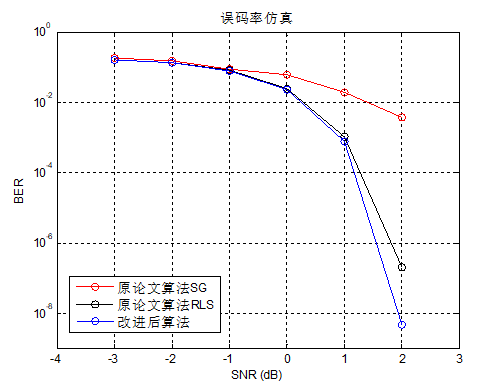对于同样的误码率值，大概提升了0.3db，

对于同样的误码率值，大概提升了0.3db，

另外当SNR<0的时候，两个性能比较接近。但是放大之后仔细看，改进后的效果还是有略微的提升。

展开全文LCMV
• matlab代码，直接应用输入输出数据辨识传递函数模型，且包含多种传递函数模型结构的辨识matlab建模
• 动力学参数辨识方案完整过程： 建立动力学模型，对动力学模型进行线化（辨识模型，得到用于动力学参数辨识的线性模型）、参数独立性分析及最小参数集、辨识轨迹优化、辨识算法构造、参数采集与处理、实验验证。...算法
• 针对非线性模型的参数估计寻优较为困难的问题，提出一种基于改进的差分进化算法的非线性系统模型参数辨识新方法。通过引入一个自适应变异率，随着迭代的进行自适应调整缩放因子，从而在初期保持种群多样性以避免早熟...差分进化算法 非线性系统 参数辨识
• RLS参数辨识，最小二乘法RLS参数辨识，最小二乘法RLS参数辨识，最小二乘法
• 此资源用来进行参数辨识，该算法为遗传算法，用此来进行辨识准确。参数辨识
• 通过对电力负荷元件进行静态故障模拟,在系统辨识原理的基础上,运用最优化理论的Lemke辨识算法,对实验故障数据进行负荷建模和参数辨识,并列出了日光灯、电风扇、空调及三者组合的静态特征参数。分析结果表明:由于电器...静态模型 参数辨识 Lemke算法matlab
•动力学参数辨识 验证流程...